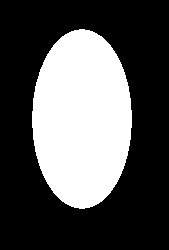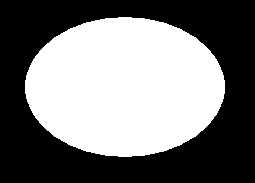Open In App
Related Articles

# fillellipse() function in C

The header file graphics.h contains fillellipse() function which draws and fills an ellipse with center at (x, y) and (x_radius, y_radius) as x and y radius of ellipse.
Syntax :

```void fillellipse(int x, int y, int x_radius,

where,
(x, y) is center of the ellipse.
```

Examples :

```Input : x = 200, y = 200,
Output :Input : x = 300, y = 250,
Output :`// C Implementation for fillellipse()``#include `` ` `// driver code``int` `main()``{``    ``// gm is Graphics mode which is``    ``// a computer display mode that``    ``// generates image using pixels.``    ``// DETECT is a macro defined in``    ``// "graphics.h" header file``    ``int` `gd = DETECT, gm;`` ` `    ``// initgraph initializes the``    ``// graphics system by loading a``    ``// graphics driver from disk``    ``initgraph(&gd, &gm, ``""``);`` ` `    ``// fillellipse function``    ``fillellipse(200, 200, 50, 90);`` ` `    ``getch();`` ` `    ``// closegraph function closes the``    ``// graphics mode and deallocates``    ``// all memory allocated by``    ``// graphics system .``    ``closegraph();`` ` `    ``return` `0;``}`
```